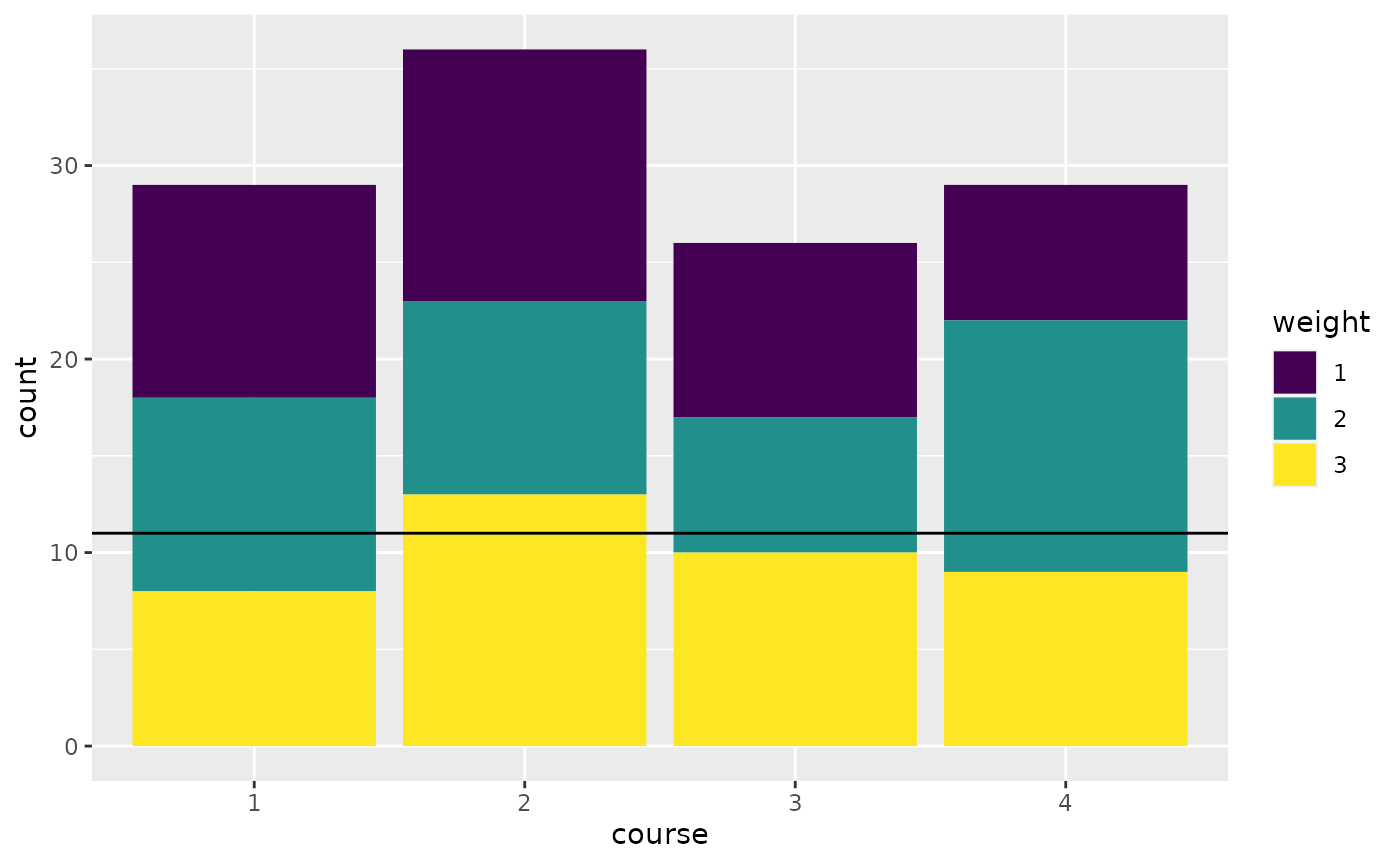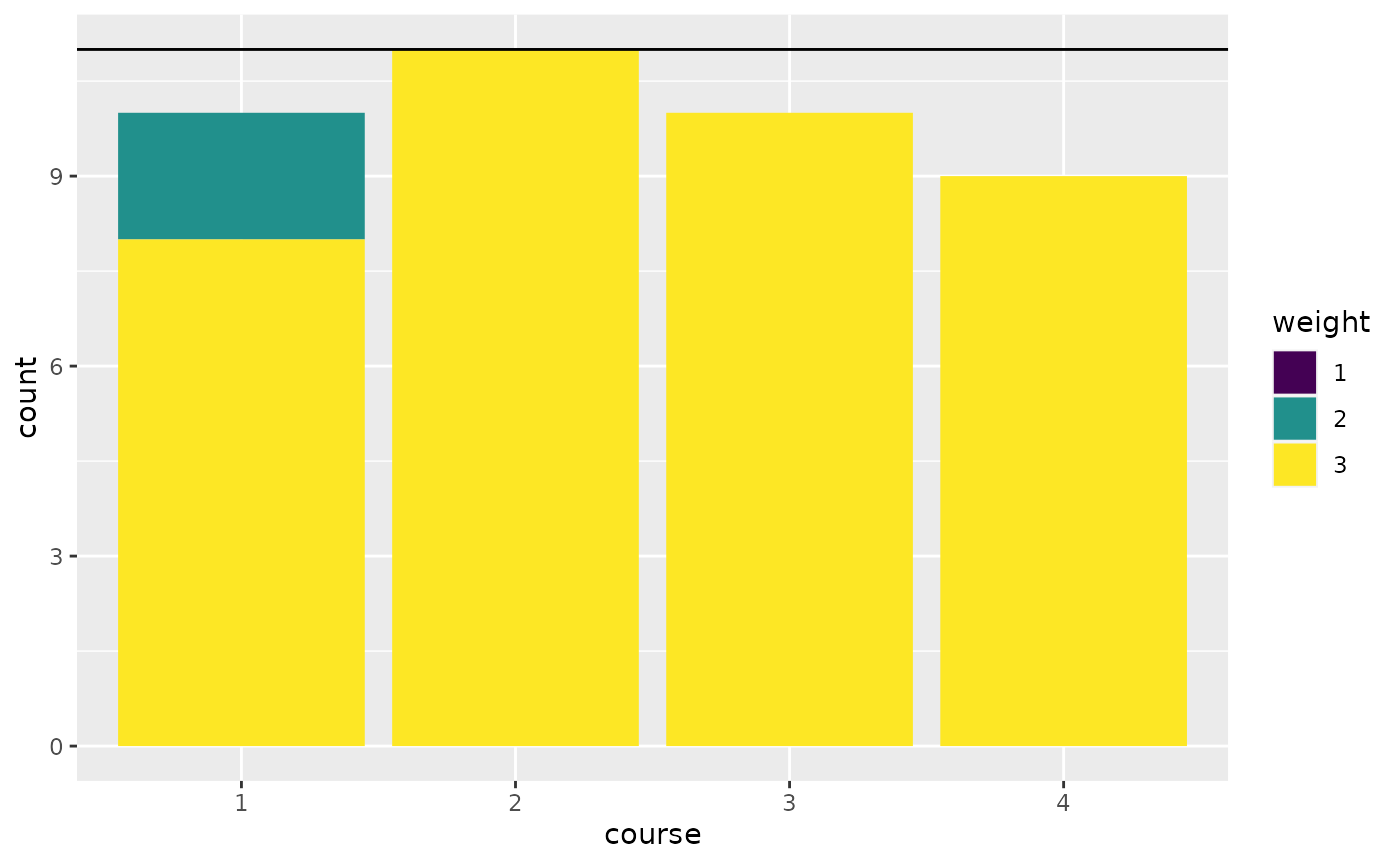## Introduction

As a real world example problem we would like to match a group of students to a set of courses with the following constraints:

• Each course has a capacity
• Every student needs to be assigned to exactly one course.
• All students have stated individual preferences on a scale from 1 to 3, where 3 is the most favorable course.

## The data

We have $$n$$ students:

n <- 40

And $$m$$ courses with equal capacity. The capacity can vary among courses though.

m <- 4
capacity <- rep.int(11, m) # all have equal capacities

In addition, each student has three preferences. To model this we have a function that gives us three courses for each student. The first component has perference 1, second 2, and third 3:

set.seed(1234)
preference_data <- lapply(seq_len(n), function(x) sample(seq_len(m), 3))
preferences <- function(student) preference_data[[student]]
preferences(1)
##  4 2 3

The last component we need is a weight functions to make the model formulation easier. This function gives us the preference weighting for a course and student pair.

# the weight of a student choosing a course
# if the course is not among the preferences, the weight is -100000
weight <- function(student, course) {
p <- which(as.numeric(course) == preferences(as.numeric(student)))
as.integer(if (length(p) == 0) {
-100000
} else {
p
})
}

Some examples:

weight(1, 3)
##  3
weight(1, 23) # this was not a choice by student 1, so we give it a big penalty
##  -100000

Let’s take a look at our random preferences. We plot the number of votes for each course grouped by the preference (1, 2, 3).

library(ggplot2)
library(purrr)
library(dplyr)
plot_data <- expand.grid(
course = seq_len(m),
weight = 1:3
) %>% rowwise() %>%
mutate(count = sum(map_int(seq_len(n), ~weight(.x, course) == weight))) %>%
mutate(course = factor(course), weight = factor(weight))
ggplot(plot_data, aes(x = course, y = count, fill = weight)) +
geom_bar(stat = "identity") +
viridis::scale_fill_viridis(discrete = TRUE) +
geom_hline(yintercept = 11)## The model

The idea is to introduce a binary variable $$x_{i, j}$$ that is $$1$$ if student $$i$$ is matched to course $$j$$. As an objective we will try to satisfy preferences according to their weight. So assigning a student to a course with preference 3 gives 3 points and so forth. The model assumes, that the total capacity of the courses is enough for all students.

Here it is in mathematical notation:

$\begin{equation*} \begin{array}{ll@{}ll} \text{max} & \displaystyle\sum\limits_{i=1}^{n}\sum\limits_{j=1}^{m}weight_{i,j} \cdot x_{i, j} & &\\ \text{subject to}& \displaystyle\sum\limits_{i=1}^{n} x_{i, j} \leq capacity_j, & j=1 ,\ldots, m&\\ & \displaystyle\sum\limits_{j=1}^{m} x_{i, j} = 1, & i=1 ,\ldots, n&\\ & x_{i,j} \in \{0,1\}, &i=1 ,\ldots, n, & j=1 ,\ldots, m \end{array} \end{equation*}$

Or directly in R:

library(ompr)
model <- MIPModel() %>%

# 1 iff student i is assigned to course m
add_variable(x[i, j], i = 1:n, j = 1:m, type = "binary") %>%

# maximize the preferences
set_objective(sum_expr(weight(i, j) * x[i, j], i = 1:n, j = 1:m)) %>%

# we cannot exceed the capacity of a course
add_constraint(sum_expr(x[i, j], i = 1:n) <= capacity[j], j = 1:m) %>%

# each student needs to be assigned to one course
add_constraint(sum_expr(x[i, j], j = 1:m) == 1, i = 1:n)
model
## Mixed integer linear optimization problem
## Variables:
##   Continuous: 0
##   Integer: 0
##   Binary: 160
## Model sense: maximize
## Constraints: 44

## Solve the model

We will use glpk to solve the above model.

library(ompr.roi)
library(ROI.plugin.glpk)
result <- solve_model(model, with_ROI(solver = "glpk", verbose = TRUE))
## <SOLVER MSG>  ----
## GLPK Simplex Optimizer, v4.65
## 44 rows, 160 columns, 320 non-zeros
##       0: obj =  -0.000000000e+00 inf =   4.000e+01 (40)
##      43: obj =  -8.999410000e+05 inf =   0.000e+00 (0)
## *   139: obj =   1.180000000e+02 inf =   0.000e+00 (0)
## OPTIMAL LP SOLUTION FOUND
## GLPK Integer Optimizer, v4.65
## 44 rows, 160 columns, 320 non-zeros
## 160 integer variables, all of which are binary
## Integer optimization begins...
## Long-step dual simplex will be used
## +   139: mip =     not found yet <=              +inf        (1; 0)
## +   139: >>>>>   1.180000000e+02 <=   1.180000000e+02   0.0% (1; 0)
## +   139: mip =   1.180000000e+02 <=     tree is empty   0.0% (0; 1)
## INTEGER OPTIMAL SOLUTION FOUND
## <!SOLVER MSG> ----

We solved the problem with an objective value of 118.

matching <- result %>%
get_solution(x[i,j]) %>%
filter(value > .9) %>%
select(i, j) %>%
rowwise() %>%
mutate(weight = weight(as.numeric(i), as.numeric(j)),
preferences = paste0(preferences(as.numeric(i)), collapse = ",")) %>% ungroup
head(matching)
## # A tibble: 6 x 4
##       i     j weight preferences
##   <int> <int>  <int> <chr>
## 1     4     1      3 4,2,1
## 2    10     1      3 3,4,1
## 3    13     1      3 3,4,1
## 4    15     1      3 4,2,1
## 5    23     1      3 3,2,1
## 6    31     1      3 3,2,1
matching %>%
group_by(weight) %>%
summarise(count = n())
## summarise() ungrouping output (override with .groups argument)
## # A tibble: 2 x 2
##   weight count
##    <int> <int>
## 1      2     2
## 2      3    38

38 students got their top preference. 2 students were assigned to their second choice and 0 students got their least preferable course.

The course assignment now looks like this:

plot_data <- matching %>%
mutate(course = factor(j), weight = factor(weight, levels = c(1, 2, 3))) %>%
group_by(course, weight) %>%
summarise(count = n()) %>%
tidyr::complete(weight, fill = list(count = 0))
## summarise() regrouping output by 'course' (override with .groups argument)
ggplot(plot_data, aes(x = course, y = count, fill = weight)) +
geom_bar(stat = "identity") +
viridis::scale_fill_viridis(discrete = TRUE) +
geom_hline(yintercept = 11)## Feedback

Do you have any questions, ideas, comments? Or did you find a mistake? Let’s discuss on Github.